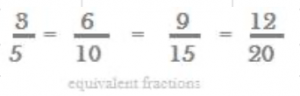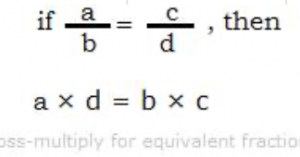Equivalent fractions are different fractions having a same value. A fraction is part of whole. i.e.

Fraction = Part/Whole = numerator/denominator

Therefore, if both of part and whole (i.e. numerator and denominator) grow equally, then the value of fraction can continue to remain same.Hence, fractions with different numerators and denominators but having a same value are called equivalent fractions. The following figure illustrates the concept of equivalent fractions. In the above figure, ABCD is a rectangle and PQRS is a circle.

{In ABCD, AC is diagonal, and in PQRS, O is center}

The area of the rectangle shaded is ½, and the area of the circle shaded is 2/4. Though ½ and 2/4 are two different fractions, still they have a same value i.e. in the respective figures a same fraction of the area is shaded. A few examples of equivalent fractions are 1/2 and 3/6, 3/4 and 6/8.

How to find equivalent fractions of given fraction? Fractions continue to have same value even when both of their numerators and denominators grow equally. Therefore, to find equivalent fractions of a given fraction: multiply or divide numerators and denominators with a same value.

## 1. Equivalent fractions of 3/5 thru multiplying areIn the equivalent fractions for 3/5, the numerator and denominator are multiplied by a same value.

6/10 = (3 × 2)/ (5 × 2)

9/15 = (3 × 3)/ (5 × 3)

12/20 = (3 × 4)/ (5 × 4)

All of the fractions 6/10, 9/15 and 12/20 have a same value as 3/5 does.

Therefore, a few equivalent fractions of 3/5 are 6/10, 9/15 and 12/20.

## 2. Equivalent fractions of 32/40 thru dividing are:

(32÷ 2)/ (40 ÷ 2) = 16/20,

(32÷ 4)/ (40 ÷ 4) = 8/10,

(32÷ 8)/ (40 ÷ 8) = 4/5

All of the fractions 16/20, 8/10 and 4/5 have a same value as 32/40 does. Therefore, a few equivalent fractions of 32/40 are 16/20, 8/10 and 4/5.

Note:

1. Addition of a same number to numerator and denominator will change the value of the fraction. 3/5 ≠ (3 + 2)/ (5 + 2), i.e. 3/5 ≠ 5/7

2. Subtraction of a same number from both numerator and denominator will also not yield equivalent fractions. 5/ 7 ≠ (5 – 2)/ (7 – 2) ≠ 3/5

How to determine if two fractions are equivalent fractions:

Consider the two fractions a/b and c/d. Cross multiply the two fractions a/b and c/d, to find if they are equivalent fractions as below.

If a/b and c/d are equivalent fractions, then a × d = b × c

Example:

Find whether the two fractions 5/9 and 30/54 are equivalent fractions.

Solution:Cross multiply the two fractions 5/9 and 30/54 as below:

5 × 54 = 9 × 30

i.e. 270 = 270.

Therefore, 5/9 and 30/54 are equivalent fractions.